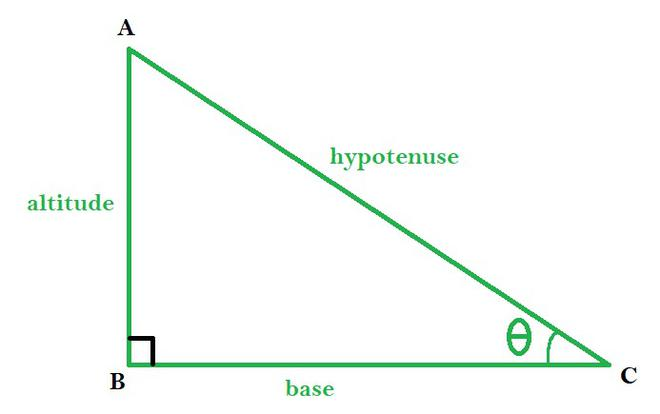# How to find an Angle in a Right-Angled Triangle?

• Last Updated : 01 Jan, 2022

Triangles are three-sided closed polygons formed by the intersection of three lines. It is encountered a lot in everyday life. It is one of the basic shapes of geometry. It has three sides, three angles, and three vertices. A Right Angled Triangle is one where one of the angles is always equal to 90°. Pythagoras Theorem is derived for Right-angled triangles, Which states that the square of the hypotenuse (the longest side) is equal to the sum of the squares of base and perpendicular.

Given the length of at least two sides of a Right-Angled triangle, we can find the value of any angle of the right-angled triangle. For this, we use various trigonometric functions like sine, cosine, tangent, cotangent, sec, and cosec. These help us to relate the angles of a right-angled triangle with its sides.

Properties

• There is a right-angle vertex among the three vertices
• The side opposite to the right-angled vertex is called the hypotenuse.
• The length of the sides follows the Pythororus theorem, which states

hypotenuse2 = base2 + altitude2

• The hypotenuse is the longest side of a right-angled triangle.
• The angles other than the right angle are acute angles since the value is less than 90o

Trigonometric functionsABC is a right-angled triangle with ∠B as right-angle

• cosθ: This gives the ratio of the base by the hypotenuse of a right-angled triangle.

cosθ = base / hypotenuse

• sinθ: This gives the ratio of altitude by the hypotenuse of a right-angled triangle.

sinθ = altitude / hypotenuse

• tanθ: It is the ratio of altitude by the base of a right-angled triangle.

tanθ = altitude / base

• cotθ: It is the inverse of tanθ
• secθ: It is the inverse of cosθ
• cosecθ: It is the inverse of sinθ

To find the angles of a right-angled triangle, we can take the trigonometric inverse of the ratio of given sides of the triangle.

Example:

If sinθ = x, then we can write

θ = sin-1x.

This returns the angle for which the sine value of the angle is x.

Similarly, there exists cos-1θ, tan-1θ, cot-1θ, sec-1θ, and cosec-1θ

### Sample Problems

Question 1. Given a right-angled triangle, with base equals 10cm and hypotenuse equals 20cm. Find the value of the base angle.

Solution:

Given, Base = 10cm

Hypotenuse = 20cm

Let, the value of the base angle be θ. We can write

cosθ = base / hypotenuse = 10/20 = 1/2

θ = cos-1(1/2) = 60o

Thus, the value of base angle is 60o.

Question 2. Find the value of angles of a right angles triangle, given that one of the acute angles is twice the other.

Solution:

Since we know the sum of all the three angles in a triangle is 180o.

Since one of the angles is 90o and one of the acute angles is twice the other, we can consider them as θ and 2θ.

So, we can write

90o + θ + 2θ = 180o

3θ = 180o – 90o

3θ = 90o

θ = 90o/3 = 30o

2θ = 2 × 30o = 60o

So, the angles are 30o, 60o, and 90o.

Question 3. Find the value of the angle of elevation of a ladder of length 5m, given that base of the ladder is at a distance of 3m from the wall.

Solution:

Since the ladder acts as a hypotenuse of a right angles triangle and base distance equals 3m, we can write

Hypotenuse = 5m

Base = 3m

Let the angle of elevation be θ. So, we can write

cosθ = Base / Hypotenuse = 3/5

θ = cos-1(3/5)

θ = 53o

Thus, the value of the angle of elevation is 53o.

Question 4. Find the value of hypotenuse, given the length of the altitude is 8m and the base angle equals 30o.

Solution:

Given, the base angle is equal to 30o and altitude equals 8m, we can apply the sine function to find the length of the hypotenuse.

sin30o = altitude / hypotenuse

hypotenuse = altitude / sin30o

Since the value of sin30o equals 1/2, we can write

hypotenuse = altitude / (1/2) = 2 × altitude

Thus, hypotenuse = 2 × 8 = 16m

Thus, the length of the hypotenuse is equal to 16m.

My Personal Notes arrow_drop_up
Recommended Articles
Page :